﻿ Multi-objective Job Shop Scheduling Using Pre-emptive Constraint ProcedurePublications are Open
Access in this journal
Article Versions
Export Article
• Normal Style
• MLA Style
• APA Style
• Chicago Style
Research Article
Open Access Peer-reviewed

### Multi-objective Job Shop Scheduling Using Pre-emptive Constraint Procedure

Jaber S. Alzahrani
American Journal of Modeling and Optimization. 2019, 7(1), 8-13. DOI: 10.12691/ajmo-7-1-2
Received March 11, 2019; Revised April 20, 2019; Accepted April 26, 2019

### Abstract

In this paper, a multi-objective job shop scheduling through the pre-emptive constraint procedure has been formulated to optimize makespan, total earliness and total tardiness. The effectiveness of the model is studied through eighteen 3J*3M and three 10J*10M problems. The model is solved using Mosel language with Xpress software.

### 1. Introduction

The aim of Job Shop Scheduling (JSS) is to allocate the required resources to perform the specific tasks in a given period to achieve great resources utilization, minimum cost including out of time penalties and other objectives. JSS problem was defined as one of the production planning functions which includes a set of hardest problems in combinatorial optimization 1.

Many single objective studies have been made 2. A MIP model for single machine scheduling with the tardiness objective has been formulated 3. Also, the flow shop and job shop problems to minimize makespan has been addressed 4, 5.

For multi-objective optimization, Becerra and C. A. Coello 6, proposed a combined procedure of a previously developed single-objective approach together with the ϵ-constraint method to provide an approximation of the Pareto front in multi-objective. M. Laumanns et al. 7, proposed some methods to approximate the Pareto set of multi-objective optimization problems by solving a sequence of constrained single-objective problems. R. T. Marler and J. S. Arora 8 presented a comprehensive study about the methods of multi-objective optimization. However, J.-F. Bérubé, M. Gendreau, and J.-Y. Potvin 9 has recently introduced an innovative solution approach, the ϵ-constraint method. R. L. A multi-objective JSS model using the preemptive objectives procedure is formulated by M. S. Al-Ashhab 10.

In electricity fields, M. Boulif and K. Atif 11 adopted a ϵ-constraint method to solve the manufacturing cell formation problem minimizing both the inter-cellular movements and the workload unbalance. M. Esmaili et al. 12 addressed the multi-objective congestion management for optimizing the congestion management cost, voltage security and dynamic security. L. Grandinetti et al. 13 described an approximate ϵconstraint-based heuristic to solve the multi-objective Undirected Capacitated Arc Routing Problem.

In goal programming, there are four models:

a) Pre-emptive Goal Programming Using Constraints

b) Archimedean Goal Programming Using Constraints

c) Pre-emptive Goal Programming Using Objective Functions

d) Archimedean Goal Programming Using Objective Functions

In this study, Pre-emptive Goal Programming Using Constraints procedure is used in which the constraints are used to construct the goals, and the goals are to minimize the violation of these constraints. When the constraints are satisfied, the goals are met.

The used procedure is illustrated through the following example which has three objectives named; goal 1, goal 2 and goal 3 as shown in Table 1.

Initially, the procedure tries to meet the first goal, which can be done with x = 5.0 and y = 1.6, but this solution does not satisfy the second goal or the third goal. Then it tries to meet the second goal while still achieving the first goal, the solution x = 6.0 and y = 0.0 will satisfy. However, this does not fulfil the third goal, so it repeats the process. On this occasion, no solution exists which meets all three 14.

In this research, a Multi-Objective Job Shop Scheduling model using Pre-emptive goal programming using constraints procedure has been formulated to optimize makespan, total earliness and total tardiness.

The following assumptions have been considered:

1) All jobs and machines are available at the beginning.

2) Recirculation of jobs is not allowed.

### 2. Model Formulation

Parameters:

N: the set of jobs;

Dj: due date of job j;

M: the set of machines;

SEQ: processing sequences.

Phj: duration times for job j on machine h;

Decision variables:

Shj: Starting time of job j on machine h;

Cj: Completion time of job j;

Ej: Earliness of job j;

Tj: Tardiness of job j.

The three objectives; makespan, total earliness, and total tardiness are declared in Equations (1- 3).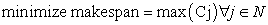(1)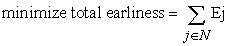(2)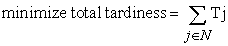(3)

Subject to conjunctive (Equation 4) and disjunction (Equation 5, 6) constraints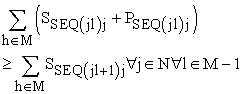(4)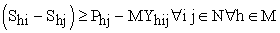(5)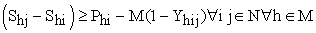(6)

### 3. Results and Analysis

In this section, the results of the developed model application are introduced and analyzed. In consequence, the model has been solved using XpressMP which operates in an Intel® Core™ i3-2310M CPU @2.10 GHz.

The model inputs of the 3Jx3M problems; processing sequences, durations and due dates, are presented in Table 2, Table 3, and Table 4 respectively. The duration and due dates are assumed to be in hours.

3.1. Study of the Makespan Worst Target Effect

Through this section, the effect of the makespan target is studied through solving three problems of makespan less, equal, and more than the maximum due date of the three jobs. The worst targets of the three objectives and their orders of the three 3Jx3M problems have been assumed as shown in Table 5.

a) Problem 1

Table 6 and Figure 1 represent the schedule and Gantt chart of the first 3Jx3M problem in which, it may be noticed that the optimal makespan of this problem is 155 hours which are better than the worst target set for the first objective of 190 hours.

• Figure 1. The Gantt chart of the first 3Jx3M problem

The resulted values of finishing time, earliness and tardiness of the three jobs of the first 3Jx3M problem are presented in Table 7.

Table 8 represents the objectives' targets, values and deviations of the first 3Jx3M problem in which it is noticed that the primary objective of minimizing the makespan have been achieved without deviation while the other two objectives have not been satisfied because of the tight target of the primary objective.

b) Problem 2

Table 9 and Figure 2 represent the schedule and Gantt chart of the second 3Jx3M problem in which, it is noticed that the optimal makespan of this problem is 210 hours which are equal to the worst target set for the first objective.

The resulted values of finishing time, tardiness and earliness of the three jobs of the second 3Jx3M problem are presented in Table 10.

Table 11 represents the objectives' targets, values and deviations of the second 3Jx3M problem in which it is noticed that all objectives have been satisfied without deviation.

c) Problem 3

The schedule and Gantt chart of the third 3Jx3M problem is similar to the same presented in Table 9 and Figure 2 in which, it may be noticed that the optimal makespan of this problem is 210 hours which is better than the worst target set for the first objective of 230 hours.

The resulted in values of finishing time, earliness and tardiness of the three jobs of the second 3Jx3M problem also similar to that shown in Table 10 and the objectives' targets, values and deviations of the third 3Jx3M problem are represented in Table 12 in which it all objectives have been satisfied without deviation.

3.2. Study of the Objectives Ordering Effect

The effect of the objectives ordering is studied in this section through eighteen problems classified as shown in Table 13. In the first group where the worst target of the makespan is assumed to be 190 min, all the six different order problems have been failed to satisfy all objectives. In the second group of 210 min worst target of the makespan, all of the six different order problems have been succeeded to fulfill all objectives without any deviations. In the third group of 230 worst targets of the makespan, all of the six different order problems have been succeeded to satisfy all objectives with a negative deviation towards the best value of the makespan.

• Figure 2. The Gantt chart the second 3Jx3M problem
3.3. 10Jx10M Problems

The aims of this section are to verify the model capability for solving large problems and to validate its results by solving three 10 jobs by 10 machines problems. The model inputs of the 10Jx10M problems; processing sequences, durations and due dates, are presented in Table 14, Table 15, and Table 16 respectively.

The worst targets of the three objectives and their orders of the three 10Jx10M problems have been assumed as shown in Table 17.

The resulted schedules Gantt charts of the three 10Jx10M problems are presented in Figure 3, Figure 4, and Figure 5.

• Figure 3. The Gantt chart of the first 10Jx10M problem
• Figure 4. The Gantt chart of the second 10Jx10M problem
• Figure 5. The Gantt chart of the third 10Jx10M problem

As shown in Table 18, the first goals are satisfied in the three cases. In the second problem, both the first and second goals are met where they are compatible.

### 4. Conclusion

In this work, a multi-objective job shop scheduling by means of the pre-emptive constraint procedure has been formulated to optimize makespan, total earliness and total tardiness. The effectiveness of the model is studied through eighteen 3J*3M and three 10J*10M problems. The models are solved using Mosel language with Xpress software.

The solving capability of the developed model has been verified through the analysis and discussion of many problems of different sizes.

It may be concluded that using the pre-emptive goal programming using constraints ensures that the achieved values of the objectives are better than the worst assigned values.

Model efficiency is verified through 21 problems. Eighteen of them are of size 3 jobs * 3 machines, and the rest are of size 10 jobs * 10 machines. All results are compared and discussed to assure the accuracy of the model.

### References

  G. Zhang, X. Shao, P. Li, and L. Gao, "An effective hybrid particle swarm optimization algorithm for multi-objective flexible job-shop scheduling problem," Computers & Industrial Engineering, vol. 56, pp. 1309-1318, 2009. In article View Article  A. Scaria, K. George, and J. Sebastian, "An Artificial Bee Colony Approach for Multi-objective Job Shop Scheduling," Procedia Technology, vol. 25, pp. 1030-1037, 2016. In article View Article  K. R. Baker and B. Keller, "Solving the single-machine sequencing problem using integer programming," Computers & Industrial Engineering, vol. 59, pp. 730-735, 2010. In article View Article  H. M. Wagner, “An integer linear‐programming model for machine scheduling,” Naval Research Logistics (NRL), vol. 6, pp. 131-140, 1959. In article View Article  A. S. Manne, “On the job-shop scheduling problem,” Operations Research, vol. 8, pp. 219-223, 1960. In article View Article  R. L. Becerra and C. A. Coello Coello, "Epsilon-constraint with an efficient cultured differential evolution," in Proceedings of the 9th annual conference companion on Genetic and evolutionary computation, 2007, pp. 2787-2794. In article View Article  M. Laumanns, L. Thiele, and E. Zitzler, "An efficient, adaptive parameter variation scheme for metaheuristics based on the epsilon-constraint method," European Journal of Operational Research, vol. 169, pp. 932-942, 2006. In article View Article  R. T. Marler and J. S. Arora, "Survey of multi-objective optimization methods for engineering," Structural and multidisciplinary optimization, vol. 26, pp. 369-395, 2004. In article View Article  J.-F. Bérubé, M. Gendreau, and J.-Y. Potvin, "An exact ϵ-constraint method for bi-objective combinatorial optimization problems: Application to the Traveling Salesman Problem with Profits," European journal of operational research, vol. 194, pp. 39-50, 2009. In article View Article  M. Al-Ashhab, "Multi-Objective Job Shop Scheduling Using a Lexicographic Procedure," International Journal of Engineering Science Invention (IJESI), vol. 7, p. 10, 2018. In article  M. Boulif and K. Atif, "An exact multiobjective epsilon-constraint approach for the manufacturing cell formation problem," in Service systems and service management, 2006 International Conference on, 2006, pp. 883-888. In article View Article  M. Esmaili, N. Amjady, and H. A. Shayanfar, "Multi-objective congestion management by modified augmented ε-constraint method," Applied Energy, vol. 88, pp. 755-766, 2011. In article View Article  L. Grandinetti, F. Guerriero, D. Laganà, and O. Pisacane, "An optimization-based heuristic for the multi-objective undirected capacitated arc routing problem," Computers & Operations Research, vol. 39, pp. 2300-2309, 2012. In article View Article  https://es.scribd.com/document/48640996/optimizer. In articleThis work is licensed under a Creative Commons Attribution 4.0 International License. To view a copy of this license, visit http://creativecommons.org/licenses/by/4.0/